# pylops.utils.seismicevents.hyperbolic2d#

pylops.utils.seismicevents.hyperbolic2d(x, t, t0, vrms, amp, wav)[source]#

Hyperbolic 2D events

Create 2d hyperbolic events given intercept time, root-mean-square velocity, and amplitude of each event

Parameters
xnumpy.ndarray

space axis

tnumpy.ndarray

time axis

t0tuple or float

intercept time at $$x=0$$ of each of hyperbolic event

vrmstuple or float

root-mean-square velocity of each hyperbolic event

amptuple or float

amplitude of each hyperbolic event

wavnumpy.ndarray

wavelet to be applied to data

Returns
dnumpy.ndarray

data without wavelet of size $$[n_x \times n_t]$$

dwavnumpy.ndarray

data with wavelet of size $$[n_x \times n_t]$$

Notes

Each event is created using the following relation:

$t_i(x) = \sqrt{t_{0,i}^2 + \frac{x^2}{v_{\text{rms},i}^2}}$

## Examples using pylops.utils.seismicevents.hyperbolic2d#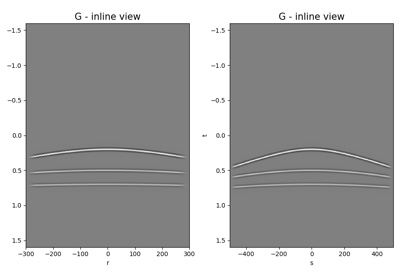Multi-Dimensional Convolution

Multi-Dimensional ConvolutionNormal Moveout (NMO) Correction

Normal Moveout (NMO) Correction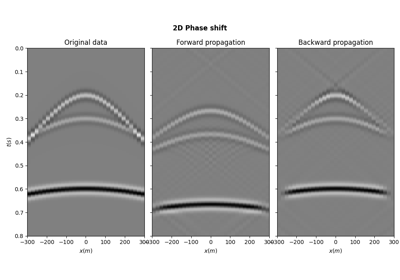PhaseShift operator

PhaseShift operator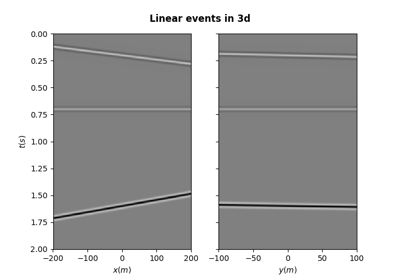Synthetic seismic

Synthetic seismic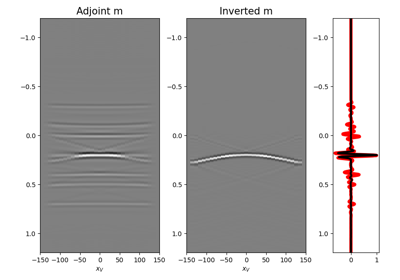09. Multi-Dimensional Deconvolution

09. Multi-Dimensional Deconvolution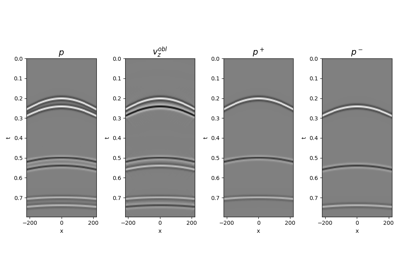14. Seismic wavefield decomposition

14. Seismic wavefield decomposition